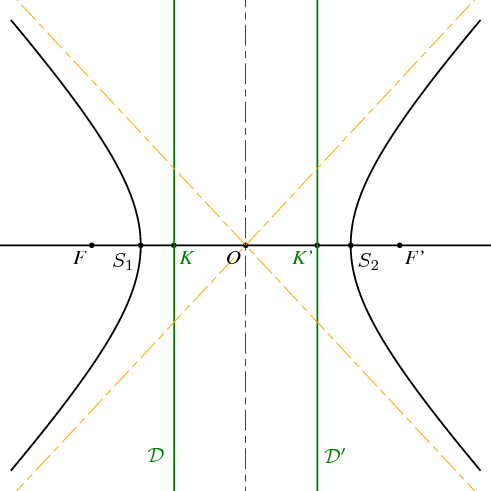Fichier 014.jps — Modifié le 11 Mars 2006 à 10 h 52Source
%% PhS
%%

50 setxunit
-3.5 3.5  setxrange
-3.5 3.5 setyrange

%%%%%%%%%%%%%%%%%%%%%%%%%%%%%%%%%%%%%%%%%%%%%%%
%% procédures
%%%%%%%%%%%%%%%%%%%%%%%%%%%%%%%%%%%%%%%%%%%%%%%

/bddroite {
8 dict begin
/kd exch def
/kg exch def
/By exch def
/Bx exch def
/B {Bx By} def
/Ay exch def
/Ax exch def
/A {Ax Ay} def
gsave
[A A B vecteur kg mulv addv A A B vecteur kd mulv addv] ligne
grestore
end
} def

%%%%%%%%%%%%%%%%%%%%%%%%%%%%%%%%%%%%%%%%%%%%%%%
%% definition d'une couleur
%%%%%%%%%%%%%%%%%%%%%%%%%%%%%%%%%%%%%%%%%%%%%%%

/coul1 {120 255 div 70 255 div 9 255 div setrgbcolor} def

%%%%%%%%%%%%%%%%%%%%%%%%%%%%%%%%%%%%%%%%%%%%%%%
%% definition des points
%%%%%%%%%%%%%%%%%%%%%%%%%%%%%%%%%%%%%%%%%%%%%%%

/a {1.5} def
/c {2.2} def
/b {c c mul a a mul sub sqrt} def
/e {c a div} def
/Kx {a e div} def
/K {Kx neg 0} def
/K+ {K 0 6 addv} def
/K- {K 0 -6 addv} def
/Kp {Kx 0} def
/Kp+ {Kp 0 6 addv} def
/Kp- {Kp 0 -6 addv} def
/O {0 0} def
/F {c neg 0} def
/Fp {c 0} def
/S1 {a neg 0} def
/S2 {a 0} def
/B {0 b} def

/xdet {settvar t t mul b b mul div 1 add sqrt a mul} def
/ydet {settvar t} def

/Mx {b 0.6 mul xdet} def
/My {b 0.6 mul ydet} def
/M {Mx My} def
/H {M Kp Kp+ orthoproj} def

/bissec {F M Fp bissectrice} def
/T {bissec Kp Kp+ interdroite} def
/U {bissec M perp Kp Kp+ interdroite} def

%%%%%%%%%%%%%%%%%%%%%%%%%%%%%%%%%%%%%%%%%%%%%%%
%% traces
%%%%%%%%%%%%%%%%%%%%%%%%%%%%%%%%%%%%%%%%%%%%%%%

1.2 setlinewidth

[O F Fp S1 S2 K Kp] points
F Fp -0.5 1.5 bddroite

b -2 mul b 2 mul settrange
{xdet} {ydet} courbeparam
b -2 mul b 2 mul settrange
{xdet neg} {ydet} courbeparam

vert
[K+ K-] ligne
[Kp+ Kp-] ligne

0.6 setlinewidth
orange
mixte
a neg b neg a b -1 2 bddroite
a  b neg a neg b -1 2 bddroite
noir

%%%%%%%%%%%%%%%%%%%%%%%%%%%%%%%%%%%%%%%%%%%%%%%
%% texte
%%%%%%%%%%%%%%%%%%%%%%%%%%%%%%%%%%%%%%%%%%%%%%%

setTimesItalic
noir
(O) O [1.5 dup] dltext
(F) F [1.5 dup] dltext
(F') Fp [1.5 dup] drtext

<latex>
$S_1$
</latex>
S1 [1.5 dup] dltexlabel

<latex>
$S_2$
</latex>
S2 [1.5 dup] drtexlabel

vert
(K) K [1.5 dup] drtext
(K') Kp [1.5 dup] dltext

<latex>
$\mathcal{D}$
</latex>
K 0 -3 addv [1.5 dup] cltexlabel

<latex>
$\mathcal{D}'$
</latex>
Kp 0 -3 addv [1.5 dup] crtexlabel